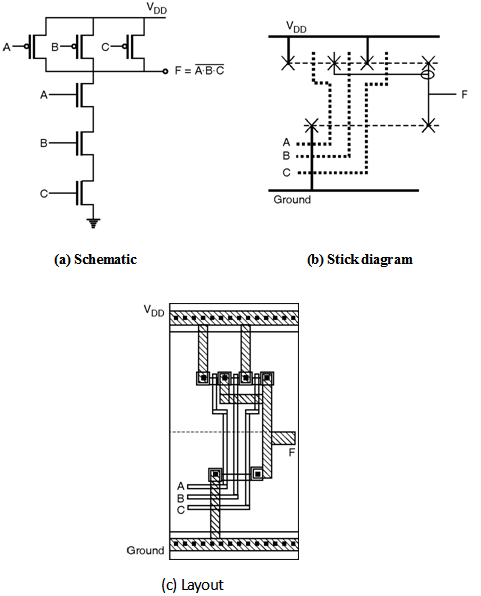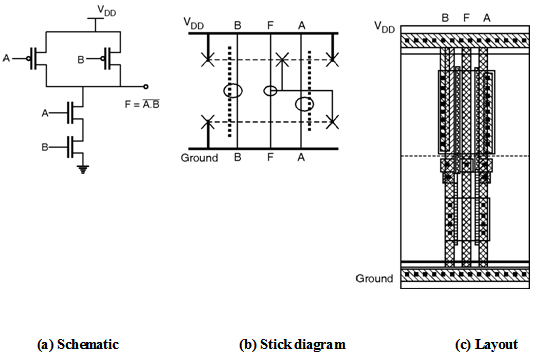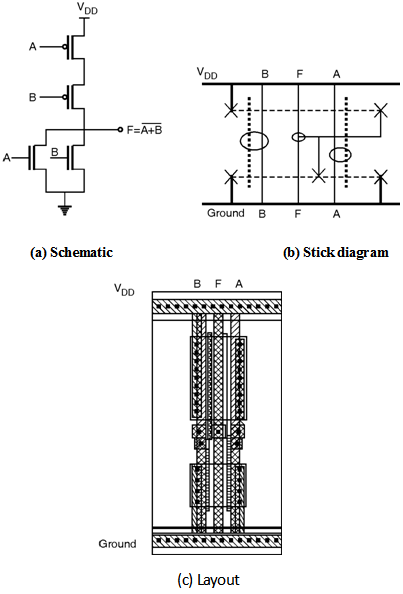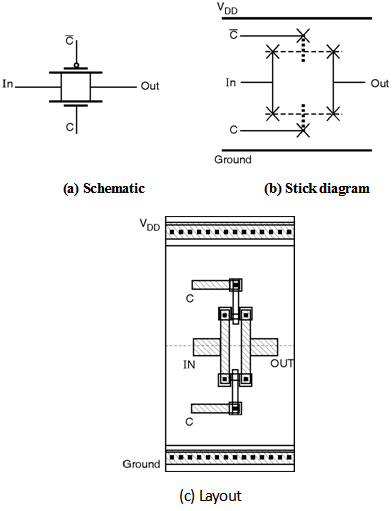Home > Digital CMOS Design > CMOS Layout Design > Layout of logic gates

CMOS-Layout-Design

Layout of Logic gates:

Three Input NAND Gate :

Figure below shows, the schematic, stick diagram and layout of three input NAND gate.Two Input NAND Gate :

Figure below shows the schematic, stick diagram and layout of two input NAND gate implemented using complementary CMOS logic.Two Input NOR Gate :

Figure below shows the schematic, stick diagram and layout of two input NOR gate implemented using complementary CMOS logic.Transmission Gate :

Figure below shows the schematic, stick diagram and layout of the transmission gate.